# SAT II Math II : Other Coordinate Geometry

## Example Questions

### Example Question #11 : Coordinate Geometry

On the coordinate plane, two lines intersect at the origin. One line passes through the point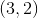; the other,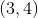Give the measures of the acute angles they form at their intersection (nearest degree).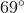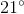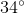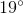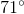Explanation:

If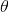is the measure of the angles that two lines with slopes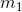and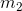form, then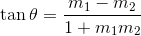,

The slopes of the lines can be found by applying the slope formula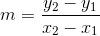using the known points.

For the first line, set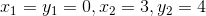: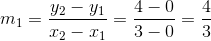The inverse tangent of this is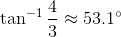,

making this the angle this line forms with the-axis.

For the second line, set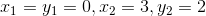: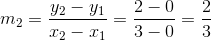The inverse tangent of this is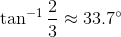making this the angle this line forms with the-axis.

Subtract:

Taking the inverse tangent: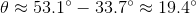.

Rounding to the nearest degree, this is.

### Example Question #12 : Coordinate Geometry

In the figure below, regular hexagon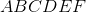has a side length of. Find the y-coordinate of point.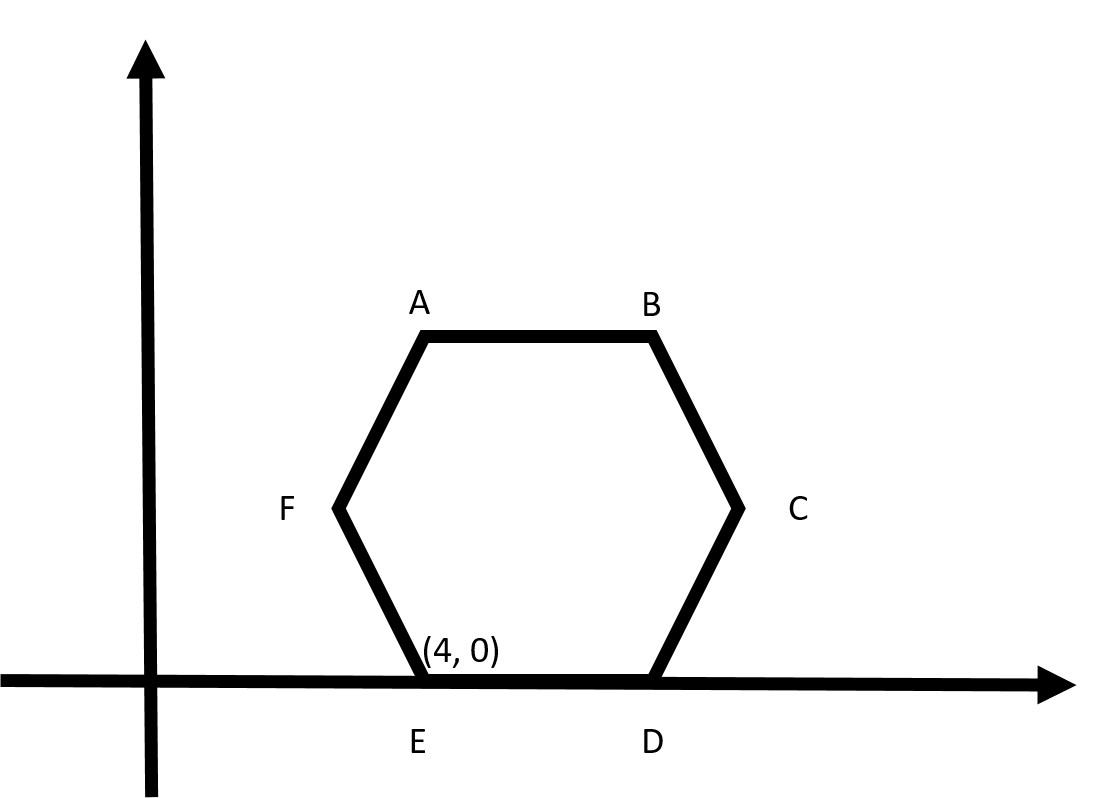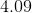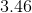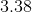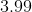Explanation:From the given information, we know that the coordinate formust be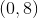.

Recall that the interior angle of a regular hexagon is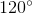. Thus, we can draw in the following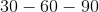triangle.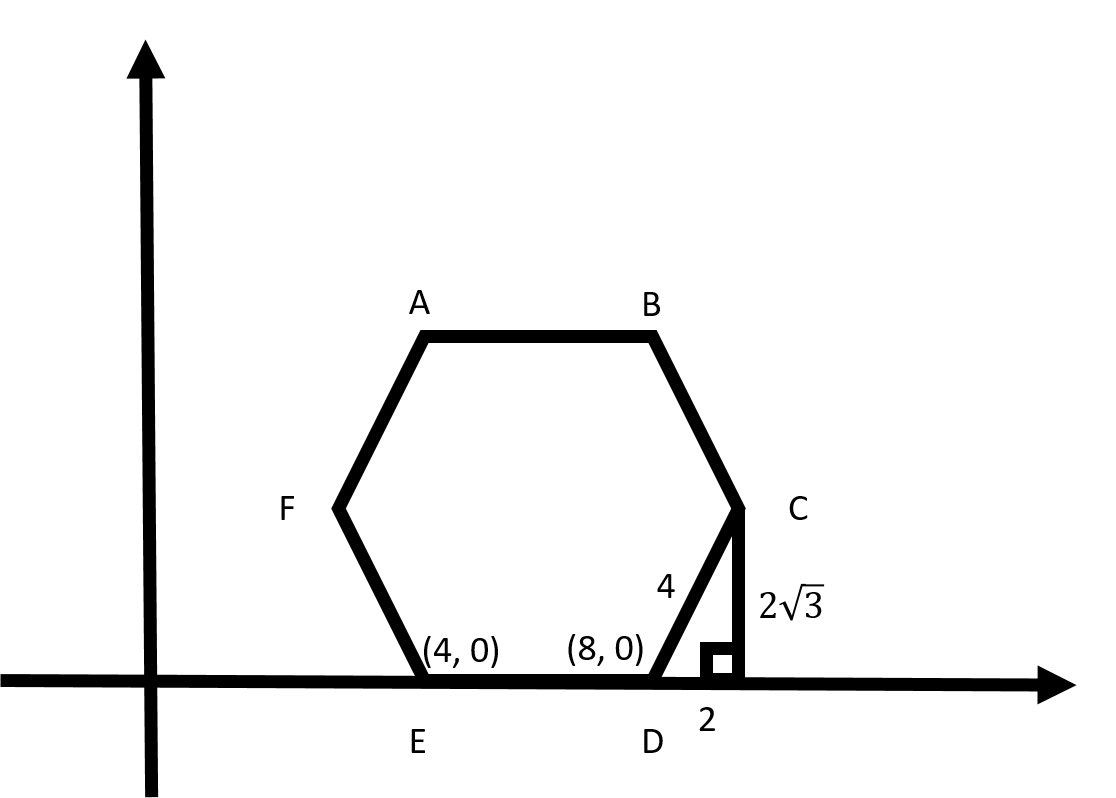Since we know that this is atriangle, we know that the sides must be as marked, in the ratio of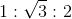. Thus, the y-coordinate ofmust be.